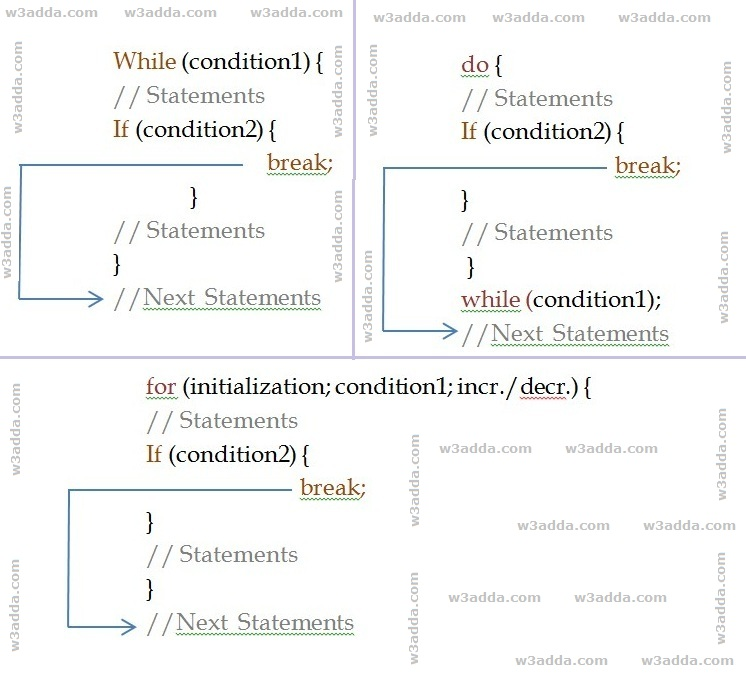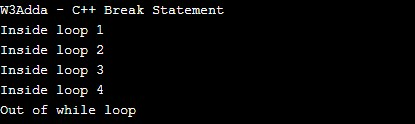# C++ Break statement

In this tutorial you will learn about the C++ Break statement and its application with practical example.

## C++ Break Statement

In C++, break statement inside any loop gives you way to break or terminate the execution of loop containing it, and transfers the execution to the next statement following the loop. It is almost always used with if..else construct.

## C++ Break Statement Flow DiagramSyntax:-

Example:-

In this above program, the variable count is initialized as 0. Then a while loop is executed as long as the variable count is less than 10. Inside the while loop, the count variable is incremented by 1 with each iteration (count = count + 1). Next, we have an if statement that checks the variable count is equal to 5, if it return TRUE causes loop to break or terminate. Within the loop there is a cout statement that will execute with each iteration of the while loop until the loop breaks. Then, there is a final cout statement outside of the while loop.

When we run this code, our output will be as follows –

Output:-In this tutorial we have learn about the C++ Break statement and its application with practical example. I hope you will like this tutorial.